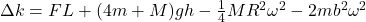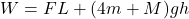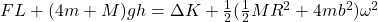## A string is wrapped around a uniform disk of mass M and radius R. Attached to the disk are four low-mass rods of radius b, each with

Question

A string is wrapped around a uniform disk of mass M and radius R. Attached to
the disk are four low-mass rods of radius b, each with a small mass m at the end. The apparatus is initially at rest. With your hand you pull the string straight up with some constant force F and while your hand is moving up a distance L, the apparatus moves downward a distance h with an angular speed ω. At this instant, determine the change in translational kinetic energy in terms of
relevant variables provided in the problem statement and g (acceleration due to gravity).

in progress 0
6 months 2021-08-09T14:56:05+00:00 1 Answers 7 views 0

Change in translational KE is given asExplanation:

As we know by work energy theorem that work done by all forces is equal to the change in kinetic energy of the system

So here we know that there are two forces acting on the system

So work done by the system of this force is equal to the change in kinetic energy

So we haveSo we haveso we have